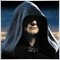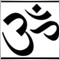# Lot size, stop loss43

Hi all,

I'm new to this, and I'm quite sure this has been discussed here already, but I can't seem to find anything helpful.

I'm trying to make an EA, and I want to set the amount I'll be trading.I know this is done by Lot size but I don't understand how its calculated.

For example if I have 10000\$ in the beginning, what lot size do I use to trade 5% of that amount?

Same thing for stop loss, how do I set stop loss to be a certain amount of money? It can be relative to the amount I'm trading.

Thank you very much in advance.

Josef1091

jozin:

For example if I have 10000\$ in the beginning, what lot size do I use to trade 5% of that amount?

Same thing for stop loss, how do I set stop loss to be a certain amount of money? It can be relative to the amount I'm trading.

This is not a coding problem, this is a basic lack of trading knowledge. Try this site ...5299

Find some MM functions Here.5299

Here I just wrote some common MM functions. You can find more descriptions Here. Let me know if you have bugs with em, and I'll try to fix. Because MM can vary differently from person to person, I wouldn't be taking any requests to add custom MM's functions, except fixing the ones below:

```//~~~~~~~~~~~~~~~~~~~~~~~~~~~~~~~~~~~~~~~~~~~~~~~~~~~~~~~~~~~~~~~~~~~~~~~~~~~~~~~~~~~~~~~~
//~~~~~~~~~~~~~~~~~~~~~~~~~~~~~~~~~~~~~~~~~~~~~~~~~~~~~~~~~~~~~~~~~~~~~~~~~~~~~~~~~~~~~~~~
//~~~~~~~~~~External_Variables:
int     Max_Slipag  =2;
int     Temp_Magic  =7;
int     Max_OrType  =9;
int     Stop__Loss  =20;
double  Int_Margin  =1.0;
double  My_FixLots  =0.7;
double  My_RiskPer  =2.0;
double  My_Mrtgale  =2.0;
//~~~~~~~~~~~~~~~~~~~~~~~~~~~~~~~~~~~~~~~~~~~~~~~~~~~~~~~~~~~~~~~~~~~~~~~~~~~~~~~~~~~~~~~~
//~~~~~~~~~~~~~~~~~~~~~~~~~~~~~~~~~~~~~~~~~~~~~~~~~~~~~~~~~~~~~~~~~~~~~~~~~~~~~~~~~~~~~~~~
//~~~~~~~~~~Constant_Variables:
double  _2Pct       =0.01;
//~~~~~~~~~~~~~~~~~~~~~~~~~~~~~~~~~~~~~~~~~~~~~~~~~~~~~~~~~~~~~~~~~~~~~~~~~~~~~~~~~~~~~~~~
//~~~~~~~~~~~~~~~~~~~~~~~~~~~~~~~~~~~~~~~~~~~~~~~~~~~~~~~~~~~~~~~~~~~~~~~~~~~~~~~~~~~~~~~~
//~~~~~~~~~~Market_Info_Variables:
int     Tick_Value;
int     Stop_Level;
int     Bkr_Freeze;
int     Margin_Req;
int     Bkr_Digits;
int     LstTicTime;
int     Acc_Cotact;
int     Order_Slip;
double  Bid_Market;
double  BkrLotSize;
double  BkrLotStep;
double  BrkMiniLot;
double  BrkMaxiLot;
double  Tick_Sizes;
double  Bkr_Points;
double  Pip_Values;
double  Order_Lots;
double  Pips_2Real;
double  Integ2Pips;
double  Int2IntPts;
//~~~~~~~~~~~~~~~~~~~~~~~~~~~~~~~~~~~~~~~~~~~~~~~~~~~~~~~~~~~~~~~~~~~~~~~~~~~~~~~~~~~~~~~~
//~~~~~~~~~~~~~~~~~~~~~~~~~~~~~~~~~~~~~~~~~~~~~~~~~~~~~~~~~~~~~~~~~~~~~~~~~~~~~~~~~~~~~~~~
//~~~~~~~~~~Global_Variables:
int     Fx__Period;
int     xOrderType;
int     xOrderSign;
double  Main_Price;
string  Fx__Symbol;
//~~~~~~~~~~~~~~~~~~~~~~~~~~~~~~~~~~~~~~~~~~~~~~~~~~~~~~~~~~~~~~~~~~~~~~~~~~~~~~~~~~~~~~~~
//~~~~~~~~~~~~~~~~~~~~~~~~~~~~~~~~~~~~~~~~~~~~~~~~~~~~~~~~~~~~~~~~~~~~~~~~~~~~~~~~~~~~~~~~
void init(){Fx__Symbol=Symbol(); Fx__Period=PERIOD_M1;}
//~~~~~~~~~~~~~~~~~~~~~~~~~~~~~~~~~~~~~~~~~~~~~~~~~~~~~~~~~~~~~~~~~~~~~~~~~~~~~~~~~~~~~~~~
//~~~~~~~~~~~~~~~~~~~~~~~~~~~~~~~~~~~~~~~~~~~~~~~~~~~~~~~~~~~~~~~~~~~~~~~~~~~~~~~~~~~~~~~~
void start(){
if(OrdersTotal()==0){
Market_Info(Fx__Symbol);
//Order_Lots=Lot_Size('b',Int_Margin);-----Example of Take_Up_X Balance.
//Order_Lots=Lot_Size('e',Int_Margin);-----Example of Take_Up_X Equity.
//Order_Lots=Lot_Size('f',My_FixLots);-----Example of Fixed Lots.
//Order_Lots=Lot_Size('n',9999999999);-----Example of Fixed None/BrokerMin.
//Order_Lots=Lot_Size('o',My_Mrtgale);-----Example of Fixed Martingale.
OrderSend(
OP-Stop__Loss*Integ2Pips,//Stop_Loss
OP+Stop__Loss*Integ2Pips,//Take_Profit Same Distance
"Order_Lots="+Order_Lots,Temp_Magic,0,Blue
);
}   }
//~~~~~~~~~~~~~~~~~~~~~~~~~~~~~~~~~~~~~~~~~~~~~~~~~~~~~~~~~~~~~~~~~~~~~~~~~~~~~~~~~~~~~~~~
//~~~~~~~~~~~~~~~~~~~~~~~~~~~~~~~~~~~~~~~~~~~~~~~~~~~~~~~~~~~~~~~~~~~~~~~~~~~~~~~~~~~~~~~~
double Lot_Size(int Switch, double Flexable){
double Ans=Flexable; double AE=AccountEquity(); double AB=AccountBalance();
//https://www.mql5.com/en/docs/standardlibrary/ExpertClasses/SampleMMClasses
/*CopyRight Ubzen*//************ Do-Not Remove My Credit....Thank You.*/
//~~~~~~~~~~~~~~~~~~~~
switch(Switch){
case 'f':   if(Switch=='f'){break;}// Fixed Lots or Help Format Floating Lots You Provide
case 'n':   if(Switch=='n'){return(BrkMiniLot);}// None or Broker Minimum Lots
case 'b':   if(Switch=='b'){Ans=(AB*Flexable*_2Pct)/Margin_Req;}//Size Takes Up *F% of My Balance
case 'e':   if(Switch=='e'){Ans=(AE*Flexable*_2Pct)/Margin_Req;}//Size Takes Up *F% of My Equity
case 'r':   if(Switch=='r'){Ans=AB*Flexable*_2Pct/Stop__Loss/Pip_Values;}//Dont Want To Lose AnyMore Than *F%
case 'o':   if(Switch=='o'){Ans=LoserLots()*Flexable;}//Progression *Like Management (Example Martingale) *Factor=2
}
if(Switch=='r' && Ans<BrkMiniLot){return(0);}
Ans=MathFloor(Ans/BkrLotStep)*BkrLotStep;
if(Ans<BrkMiniLot){Ans=BrkMiniLot;}
if(Ans>BrkMaxiLot){Ans=BrkMaxiLot;} return(Ans);
//~~~~~~~~~~~~~~~~~~~~
}
//~~~~~~~~~~~~~~~~~~~~~~~~~~~~~~~~~~~~~~~~~~~~~~~~~~~~~~~~~~~~~~~~~~~~~~~~~~~~~~~~~~~~~~~~
//~~~~~~~~~~~~~~~~~~~~~~~~~~~~~~~~~~~~~~~~~~~~~~~~~~~~~~~~~~~~~~~~~~~~~~~~~~~~~~~~~~~~~~~~
double LoserLots(){
for(int i=OrdersHistoryTotal()-1; i>=0; i--){
if(OrderSelect(i, SELECT_BY_POS, MODE_HISTORY)
&& OrderMagicNumber()==Temp_Magic
&& OrderSymbol()==Fx__Symbol
&& OrderProfit()<0){return(OrderLots());}}}
//~~~~~~~~~~~~~~~~~~~~~~~~~~~~~~~~~~~~~~~~~~~~~~~~~~~~~~~~~~~~~~~~~~~~~~~~~~~~~~~~~~~~~~~~
//~~~~~~~~~~~~~~~~~~~~~~~~~~~~~~~~~~~~~~~~~~~~~~~~~~~~~~~~~~~~~~~~~~~~~~~~~~~~~~~~~~~~~~~~
void Market_Info(string Symb){
//~~~~~~~~~~~~~~~~~~~~~~~~
Bid_Market=     MarketInfo(Symb,MODE_BID);
Tick_Value=     MarketInfo(Symb,MODE_TICKVALUE);
Tick_Sizes=     MarketInfo(Symb,MODE_TICKSIZE);
Stop_Level=     MarketInfo(Symb,MODE_STOPLEVEL);
BkrLotSize=     MarketInfo(Symb,MODE_LOTSIZE);
BkrLotStep=     MarketInfo(Symb,MODE_LOTSTEP);
BrkMiniLot=     MarketInfo(Symb,MODE_MINLOT);
BrkMaxiLot=     MarketInfo(Symb,MODE_MAXLOT);
Bkr_Freeze=     MarketInfo(Symb,MODE_FREEZELEVEL);
Margin_Req=     MarketInfo(Symb,MODE_MARGINREQUIRED);
Bkr_Digits=     MarketInfo(Symb,MODE_DIGITS);
Bkr_Points=     MarketInfo(Symb,MODE_POINT);
LstTicTime=     MarketInfo(Symb,MODE_TIME);
Acc_Cotact=     MarketInfo(Symb,MODE_MARGININIT);
//~~~~~~~~~~~~~~~~~~~~Integ2Pips:
Integ2Pips=     Bkr_Points;         Int2IntPts=1;
if(Bkr_Digits==2 || Bkr_Digits==3){ Pips_2Real=100;}
if(Bkr_Digits==4 || Bkr_Digits==5){ Pips_2Real=10000;}
if(Bkr_Digits==3){Integ2Pips=0.01;  Int2IntPts=10;}
if(Bkr_Digits==5){Integ2Pips=0.0001;Int2IntPts=10;}
//~~~~~~~~~~~~~~~~~~~~Pip_Values
Pip_Values=Tick_Value / (Tick_Sizes*Pips_2Real);
//~~~~~~~~~~~~~~~~~~~~Slippage
Order_Slip=Max_Slipag * Int2IntPts;
//~~~~~~~~~~~~~~~~~~~~Main_Price
//~~~~~~~~~~~~~~~~~~~~
}
//~~~~~~~~~~~~~~~~~~~~~~~~~~~~~~~~~~~~~~~~~~~~~~~~~~~~~~~~~~~~~~~~~~~~~~~~~~~~~~~~~~~~~~~~
//~~~~~~~~~~~~~~~~~~~~~~~~~~~~~~~~~~~~~~~~~~~~~~~~~~~~~~~~~~~~~~~~~~~~~~~~~~~~~~~~~~~~~~~~```20430

jozin:

I'm trying to make an EA, and I want to set the amount I'll be trading.I know this is done by Lot size but I don't understand how its calculated.

For example if I have 10000\$ in the beginning, what lot size do I use to trade 5% of that amount?

Same thing for stop loss, how do I set stop loss to be a certain amount of money? It can be relative to the amount I'm trading.

You place the stop loss where it means the reason for taking the trade is no longer valid. For example, assuming a hammer means market bottom you put the SL x pips below the hammer low.

Your risk is then order (open price - SL) * change per point * lot size.

On a USD account with a pair xxxUSD, change per pip is \$10/lot. If your SL is 10 pips below (including the spread) you're risking \$100/lot (10 pips * \$10/pip/lot.) If your SL is 100 pips below you're risking \$1000/lot.

For other combinations the exchange rates become involved in the change per point, e.g. USD with EURJPY.

When you open one or multiple orders (multiple charts) the available margin at worse case becomes a factor to avoid a margin call. Available margin is not sufficient.

See my code for DeltaValuePerLot() and Lotsize(risk)43

Thank you guys for your quick and great responce. I'll now try to go through all the information and code you posted, and hopefully things will be little bit more clear to me:)

Josef